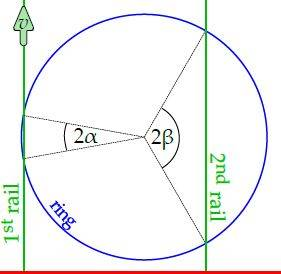# Mechanics Problem: Finding the speed at the center of a ring

## Homework Statement

A homogeneous ring lays horizontally on two identical parallel rails. The first rail moves parallel to itself, with a constant speed v; the second rail is at rest. The angular distance between the ring-rail contact points, as seen from the center of the ring, is 2α for the first rail, and 2β for the second rail, see figure. Assuming that α << 1 and β = π/3, find the speed of the center of the ring.(and also, the first rail intersects the ring at two points)

## Homework Equations

τ (torque) = F x r

## The Attempt at a Solution

I wasn't quite sure where to start but I thought I might be able to use a torque balance to find the frictional forces, though I'm not sure where to go from there, or whether I am actually taking the correct approach.